# The speed of a 3-phase, 440 V, 50 Hz induction motor is to be controlled over a wide range from zero speed to 1.5 time the rated speed using a 3-phase voltage source inverter. It is desired to keep the flux in the machine constant in the constant torque region by controlling the terminal voltage as the frequency changes. The inverter output voltage vs frequency characteristic should be

1.

2.

3.

4.

4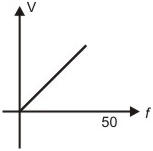Explanation :
No Explanation available for this question

# For a given sinusoidal input voltage,the voltage waveform at point P of the clamper circuit shown in figure will be

1.

2.

3.

4.

4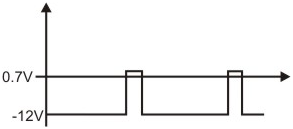Explanation :
No Explanation available for this question

# A single-phase half wave uncontrolled converter circuit is shown in figure. A 2 - winding transformer is used at the input for isolation. Assuming the load current to be constant and v = Vm sin ωt, the current waveform through diode D2 will be

1.

2.

3.

4.

4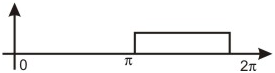Explanation :
No Explanation available for this question

# x [n] = 0; n < - 1, n > 0, x[- 1] = -1, x = 2 is the input and y [n] = 0; n < - 1, n > 2, y[- 1] = - 1 = y, y = 3, y  = -2 is the output of a discrete-time LTIsystem.The system impulse response h[n] will be

1.  h[n] = 0; n < 0, n > 2, h = 1, h = h = - 1

2.  h[n] = 0; n < -1, n > 1, h[-1] = 1, h = h = 2

3.  h[n] = 0; n < 0, n > 3, h = -1, h = 2, h = 1

4.  h[n] = 0; n < - 2, n > 1, h[- 2] = h = h[-1] = - h = 3

4

h[n] = 0; n < 0, n > 2, h = 1, h = h = - 1

Explanation :
No Explanation available for this question

# The expression V=(1 - h/H)2 dh for the volume of a cone is equal to

1.  (1 - h/H)2 dr

2.  (1 - h/H)2 dh

3.  H(1 - r/R) dh

4.  H (1 - (r/R))2 dr

4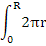H (1 - (r/R))2 dr

Explanation :
No Explanation available for this question

# The discrete-time signal x[n] ↔ X(z) = ,  where ↔ denotes a transform-pair relationship, is orthogonal to the signal

1.  y1[n] ↔ Y1(z) =

2.  y2[n] ↔ Y2(z) =

3.  y3[n] ↔ Y3(z) =

4.  y4[n] ↔ Y4(z) = 2z-4 + 3z-2 + 1

4

y1[n] ↔ Y1(z) =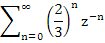Explanation :
No Explanation available for this question

# A surface S (x, y) = 2x + 5y - 3 is integrated once over a path consisting of the points that satisfy (x + 1)2 + (y - 1)2 = √2. The integral evaluates to

1.  17√2

2.  17/√2

3.  √(2)/17

4.  0

4

0

Explanation :
No Explanation available for this question

# A continuous-time system is described by y(t) = e - | x(t) |, where y (t) is the output and x (t) is the input y (t) is bounded

1.  only when x (t) is bounded

2.  only when x (t) is non-negative

3.  only for t ≥ 0 if x (t) is bounded for t ≥ 0

4.  even when x (t) is not bounded

4

even when x (t) is not bounded

Explanation :
No Explanation available for this question

# The running integrator given by y (t) =

1.  has no finite singularities in its double sided Laplace Transform Y (s)

2.  produces a bounded output for every causal bounded input

3.  produces a bounded output for every anticausal bounded input

4.  has no finite zeroes in its double sided Laplace Transform Y (s)

4

produces a bounded output for every causal bounded input

Explanation :
No Explanation available for this question

# Two fair dice are rolled and the sum r of the numbers turned up is considered

1.  Pr (r > 6) = 1/6

2.  Pr (r/3 is an integer) = 5/6

3.  Pr (r = 8 | r/4 is an integer) = 5/9

4.  Pr (r = 6 | r/5 is an integer) = 1/18

4